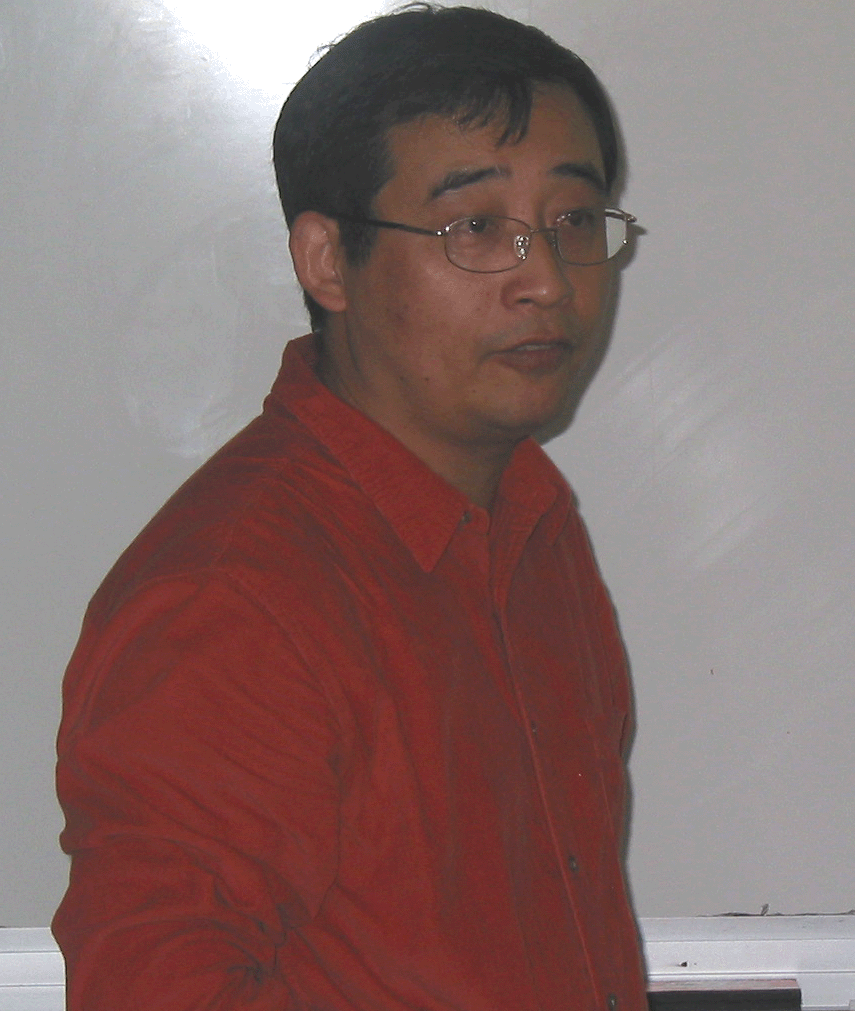[ Search | Site Map | Contact ]##### High Frequency Wave PropagationCSIC Building (#406), Seminar Room 4122.
Directions: home.cscamm.umd.edu/directions

###Hamiltonian-preserving Schemes for the Liouville Equation with Discontinuous Potentials

Shi Jin

Deptartment of Mathematics at University of Wisconsin

Abstract:  When numerically solving the Liouville equation with a discontinuous potential, one faces the problem of severe time step restriction, and the inconsistency to the constant Hamiltonian which is related to the problem of how the weak solution should be defined for such linear hyperbolic equations with singular coefficients. In this talk, we present a class of Hamiltonian-preserving schemes that are able to overcome these numerical deficiencies. The key idea is to build into the numerical flux the behavior of a classical particle at a potential barrier. We establish the stability theory of these new schemes, and analyze their numerical accuracy. Numerical experiments are carried out to verify the theoretical results. This method can also be applied to the level set methods for the computations of multivalued physical observables in the semiclassical limit of the linear Schrödinger equation with a discontinuous potential. For wave equations with discontinuous local speeds, this leads to numerical schemes consistent with Snell's Law of Refraction.

[LECTURE SLIDES]UM Home | Directories | Search | Admissions | Calendar | Web Accessibility Maintained by CSCAMM Direct questions and comments to webmaster@cscamm.umd.edu CSCAMM is part of the College of Computer, Mathematical & Natural Sciences (CMNS)